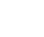# Basic math symbols

Symbol
Symbol Name
Meaning / definition
Example
=
equals sign
equality
5 = 2+3
not equal sign
inequality
5 ≠ 4
>
strict inequality
greater than
5 > 4
<
strict inequality
less than
4 < 5
inequality
greater than or equal to
5 ≥ 4
inequality
less than or equal to
4 ≤ 5
( )
parentheses
calculate expression inside first
2 × (3+5) = 16
[ ]
brackets
calculate expression inside first
[(1+2)*(1+5)] = 18
+
plus sign
1 + 1 = 2
minus sign
subtraction
2 − 1 = 1
±
plus – minus
both plus and minus operations
3 ± 5 = 8 and -2
minus – plus
both minus and plus operations
3 ∓ 5 = -2 and 8
*
asterisk
multiplication
2 * 3 = 6
×
times sign
multiplication
2 × 3 = 6
multiplication dot
multiplication
2 ∙ 3 = 6
÷
division sign / obelus
division
6 ÷ 2 = 3
/
division slash
division
6 / 2 = 3
horizontal line
division / fractionmod
modulo
remainder calculation
7 mod 2 = 1
.
period
decimal point, decimal separator
2.56 = 2+56/100
a^b
caret
exponent
2 ^ 3= 8
%
percent
1% = 1/100
10% × 30 = 3